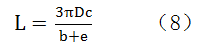Application and dosage calculation of wire harness tape

13/08/2021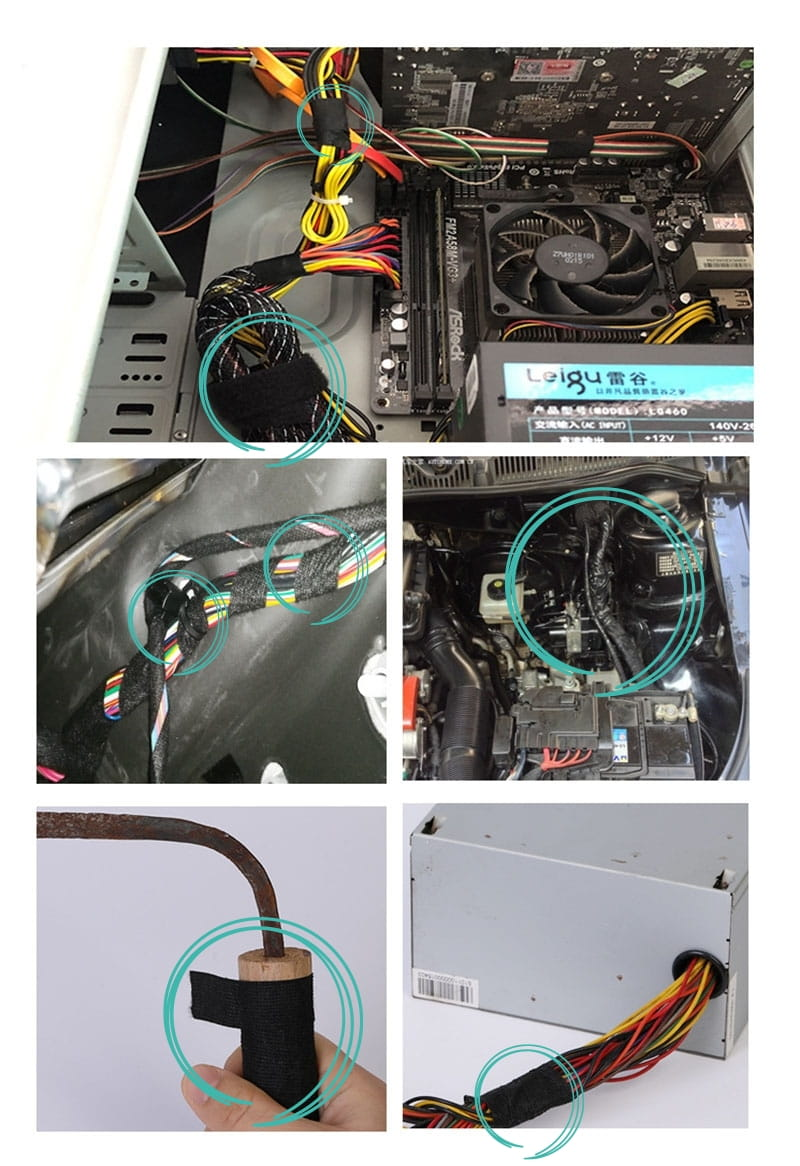Commonly used for winding wire harnesses in automobiles to reduce noise, also used for fixing wires in homes, fixing wires in computer cases, etc.

Calculation formula of tape winding length in wire harness processing

There are three commonly used tape winding methods: floral winding, dense winding and dot winding.

1. The tape is wrapped around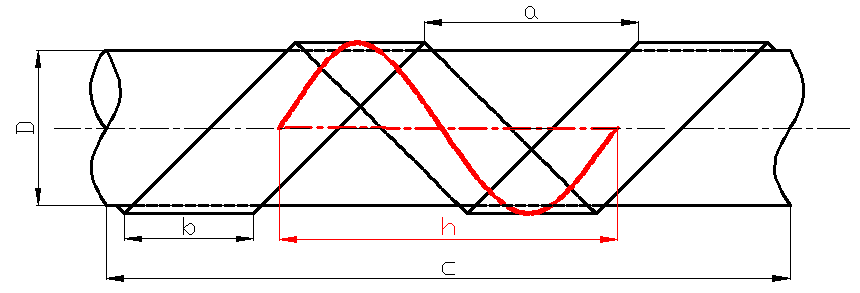Take the unit spiral length of the spiral formed by winding the tape to form a sine curve (red)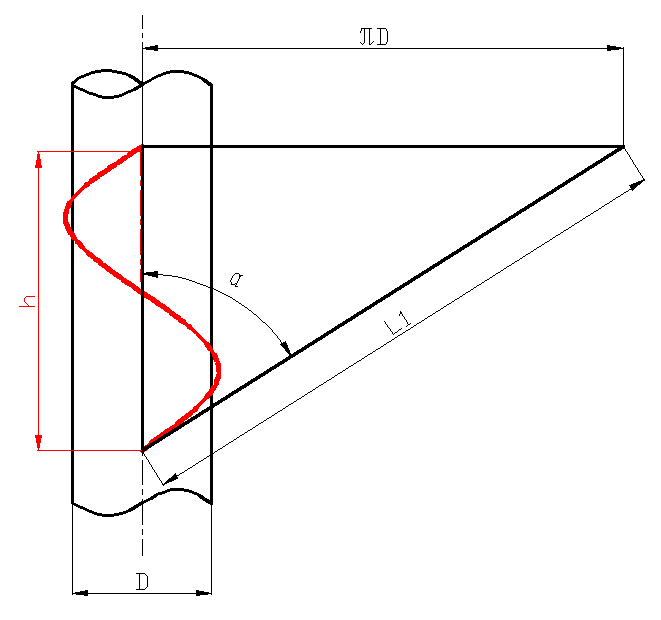Figure 2: Schematic diagram of trigonometric function relationship

The tape width used in production is 19mm and 25mm, and the formula is now derived from 19mm, that is, b=19mm, the interlacing interval is a=25mm, the sine chord length is h=a+b=44mm, and the harness diameter D=100mm , The harness length c=1000mm.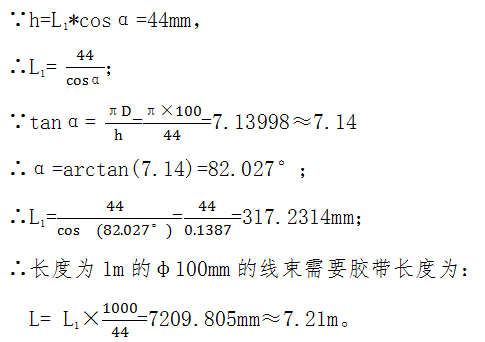Combining the above calculation processes, the calculation formula for the theoretical consumption length of tape entanglement is obtained as: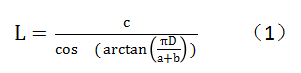2. Tape 50% overlapped and tightly wound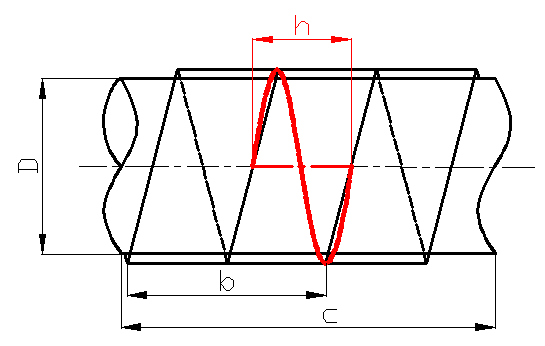50% overlapping and close wrapping is different from flower wrapping h, h=b/2;
The derivation process is the same as that of floral wrapping, and the calculation formula for the consumed length of 50% overlapping close wrapping theory is: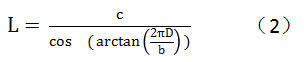※The above two tape winding methods have been verified by actual operation, and the correction coefficient A=1.02 of the actual consumed length compared to the theoretical consumed length is obtained.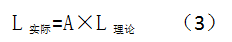Formula for calculating the actual length of tape required for wrapping: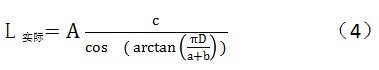The formula for calculating the actual length of tape required for 50% close wrapping: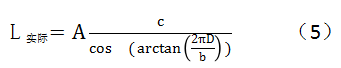3. Point the tape around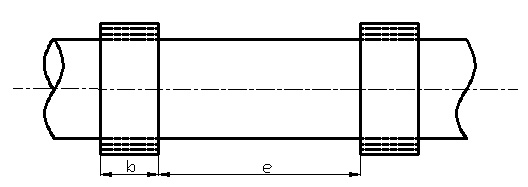The tape point winding requires 3 turns. Because the thickness of the tape is only 0.1~0.28mm, the winding thickness can be ignored, and the tape point winding interval is e.
∵The length of tape required to wrap a single point is: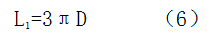∴The number of winding points in the wire harness with length c is: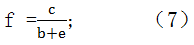∴The required tape length for the wire harness with the spread length c is: